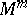# Contact surgery

A special type of surgery on a (strict) contact manifold $( M ^ { 2 n - 1 } , \xi )$ (i.e. a smooth manifold admitting a (strict) contact structure $\xi = \operatorname{ker} \alpha$, where $\alpha$ is a $1$-form satisfying $\alpha \wedge ( d \alpha ) ^ { n - 1 } \neq 0$), which results in a new contact manifold.

In topological terms, surgery ondenotes the replacement of an embedded copy of $S ^ { k } \times D ^ { m - k }$, a tubular neighbourhood of an embedded $k$-sphere with trivial normal bundle, by a copy of $D ^ { k + 1 } \times S ^ { m - k - 1 }$, with the obvious identification along the boundary $S ^ { k } \times S ^ { m - k - 1 }$. Alternatively, one can attach a $( k + 1 )$-handle $D ^ { k + 1 } \times D ^ { m - k }$ along $S ^ { k } \times D ^ { m - k }$ to a manifold $W ^ { m + 1 }$ with boundary, and the new boundary will be the result of performing surgery on.

As shown by Y. Eliashberg [a2] and A. Weinstein [a11], contact surgery is possible along spheres which are isotropic submanifolds (cf. also Isotropic submanifold) of $( M , \xi )$ and have trivial normal bundle. The choice of framing, i.e. trivialization of the normal bundle, for which contact surgery is possible is restricted.

A contact manifold $( M , \xi = \operatorname { ker } \alpha )$ may be regarded as the strictly pseudo-convex boundary of an almost-complex (in fact, symplectic) manifold $W = ( M \times ( 0,1 ] , J )$ such that $\xi$ is given by the $J$-invariant subspace of the tangent bundle $T M$. Contact surgery on $M$ can then be interpreted as the attaching of an almost-complex or symplectic handle to $W$ along $M$, and the framing condition for $n > 2$ is given by requiring the almost-complex structure on $W$ to extend over the handle. For $n = 2$ the situation is more subtle, see [a2], [a5]. Weinstein formulates his construction in terms of symplectic handle-bodies, Eliashberg (whose results are somewhat stronger) in terms of $J$-convex Morse functions on almost-complex manifolds (cf. also Almost-complex structure; Morse function).

A Stein manifold of real dimension $2 n$ has the homotopy type of an $n$-dimensional CW-complex, cf. [a8], p. 39. Eliashberg uses his construction to show that for $n > 2$ this is indeed the only topological restriction on a Stein manifold, that is, if $W$ is a $2 n$-dimensional smooth manifold with an almost-complex structure $J$ and a proper Morse function $\varphi$ with critical points of Morse index at most $n$, then $J$ is homotopic to a genuine complex structure $J ^ { \prime }$ such that $\varphi$ is $J ^ { \prime }$-convex and, in particular, $( W , J ^ { \prime } )$ is Stein.

The usefulness of contact surgery in this and other applications rests on the fact that there is an $h$-principle for isotropic spheres. This allows one to replace a given embedding $\iota : S ^ { k } \rightarrow ( M ^ { 2 n - 1 } , \xi )$ by an isotropic embedding $\iota_0$ (for $k \leq n - 1$) that is isotopic to the initial one, provided only an obvious necessary bundle condition is satisfied: If $\iota_0$ is an isotropic embedding, then its differential $T _ { \iota 0 }$ extends to a complex bundle monomorphism $T S ^ { k } \otimes \mathbf{C} \rightarrow \xi$, where $\xi$ inherits a complex structure from the (conformal) symplectic structure $d \alpha |_\xi$. The relevant $h$-principle says that, conversely, the existence of such a bundle mapping coveringis sufficient forto be isotopic to an isotropic embedding $\iota_0$.

This allows one to use topological structure theorems, such as Barden's classification of simply-connected $5$-manifolds [a1], to construct contact structures on a wide class of higher-dimensional manifolds, see [a3].

In dimension $3$ ($n = 2$) there is a different notion of contact surgery, due to R. Lutz and J. Martinet [a7]; it allows surgery along $1$-spheres embedded transversely to a contact structure $\xi$. This was used by Lutz and Martinet to show the existence of a contact structure on any closed, orientable $3$-manifold and in any homotopy class of $2$-plane fields. For applications of other topological structure theorems (such as branched coverings or open book decompositions, cf. also Open book decomposition) to the construction of contact manifolds, see [a4] and references therein.

Other types of surgery compatible with some geometric structure include surgery on manifolds of positive scalar curvature ([a6], [a9]) and surgery on manifolds of positive Ricci curvature ([a10], [a12]).

How to Cite This Entry:
Contact surgery. Encyclopedia of Mathematics. URL: http://encyclopediaofmath.org/index.php?title=Contact_surgery&oldid=50645
This article was adapted from an original article by H. Geiges (originator), which appeared in Encyclopedia of Mathematics - ISBN 1402006098. See original article